# Olympiad for Class 1 Olympiad Test Level 2: Numbers’ Magic- 2

Test Description

## 10 Questions MCQ Test General Knowledge Olympiad for Class 1 | Olympiad for Class 1 Olympiad Test Level 2: Numbers’ Magic- 2

 1 Crore+ students have signed up on EduRev. Have you?
Olympiad for Class 1 Olympiad Test Level 2: Numbers’ Magic- 2 - Question 1

### Who among the following is holding maximum number?

Detailed Solution for Olympiad for Class 1 Olympiad Test Level 2: Numbers’ Magic- 2 - Question 1

2 tens, 2 ones = 22
3 tens, 3 ones = 33
3 tens, 2 ones = 32
2 tens, 1 ones = 21
33 > 32 > 22 > 21

Olympiad for Class 1 Olympiad Test Level 2: Numbers’ Magic- 2 - Question 2

### How many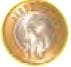Rs. 30?

Detailed Solution for Olympiad for Class 1 Olympiad Test Level 2: Numbers’ Magic- 2 - Question 2

1 coin = Rs.10
Rs10 + Rs. 10 + Rs. 10 = Rs. 30

Olympiad for Class 1 Olympiad Test Level 2: Numbers’ Magic- 2 - Question 3

### Select the incorrect match.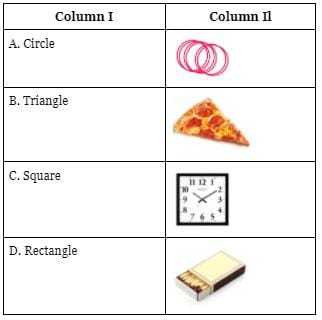Detailed Solution for Olympiad for Class 1 Olympiad Test Level 2: Numbers’ Magic- 2 - Question 3

The shape given in column b is rectangular.

Olympiad for Class 1 Olympiad Test Level 2: Numbers’ Magic- 2 - Question 4

There is/are ______ more circle(s) than triangles in the given figure.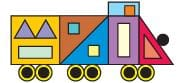Detailed Solution for Olympiad for Class 1 Olympiad Test Level 2: Numbers’ Magic- 2 - Question 4

Total number of circles = 13
Total number of triangles = 6
13 - 6 = 7

Olympiad for Class 1 Olympiad Test Level 2: Numbers’ Magic- 2 - Question 5

Which of the following is heavier than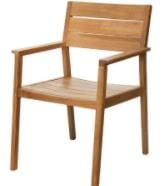?

Detailed Solution for Olympiad for Class 1 Olympiad Test Level 2: Numbers’ Magic- 2 - Question 5

A bed is larger in size than a chair.

Olympiad for Class 1 Olympiad Test Level 2: Numbers’ Magic- 2 - Question 6

Arange the following pencils from the longest to the shortest.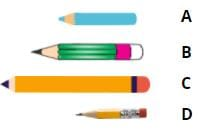Detailed Solution for Olympiad for Class 1 Olympiad Test Level 2: Numbers’ Magic- 2 - Question 6

The Correct order is C, B, A,  D.

Olympiad for Class 1 Olympiad Test Level 2: Numbers’ Magic- 2 - Question 7

______ is fifth from right hand.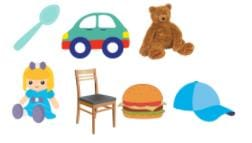Detailed Solution for Olympiad for Class 1 Olympiad Test Level 2: Numbers’ Magic- 2 - Question 7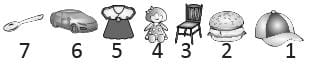Olympiad for Class 1 Olympiad Test Level 2: Numbers’ Magic- 2 - Question 8

Complete the number pattern given below: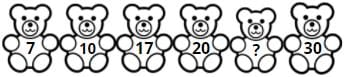The missing number is ______.

Detailed Solution for Olympiad for Class 1 Olympiad Test Level 2: Numbers’ Magic- 2 - Question 8

7 + 3 = 10
10 + 7 = 17
17 + 3 = 20
20 + 7 = 27
27 + 3 = 30

Olympiad for Class 1 Olympiad Test Level 2: Numbers’ Magic- 2 - Question 9

Find the missing figure in the given figure pattern.Detailed Solution for Olympiad for Class 1 Olympiad Test Level 2: Numbers’ Magic- 2 - Question 9

Pattern: Triangle - Square - Circle - Rectangle

Olympiad for Class 1 Olympiad Test Level 2: Numbers’ Magic- 2 - Question 10

How many more teddies should be crossed (x) to show 5 uncrossed teddies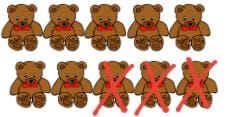Detailed Solution for Olympiad for Class 1 Olympiad Test Level 2: Numbers’ Magic- 2 - Question 10

Total no. of teddies is 10. To show 5 uncrossed teddies, there should be 5 crossed teddies. 3 are already crossed. 2 more needs to be crossed as 2 + 3 = 5.

## General Knowledge Olympiad for Class 1

20 docs|39 tests
 Use Code STAYHOME200 and get INR 200 additional OFF Use Coupon Code